## How to Simplify Fractions?

Practice Unlimited Questions

#### What is meant by simplifying a fraction?

Simplifying a fraction means writing its equivalent fraction in a simpler form.

#### How do you simplify a fraction?

To simplify a fraction, divide its numerator and denominator by the same number. Keep dividing numerator and denominator till they have a common factor. Once there are no more common factors between numerator and denominator, the fraction cannot be further simplified.

#### 1. Write 6 12 in its simplest form.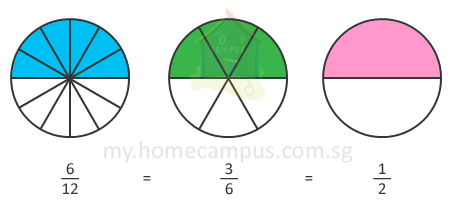A short-cut way to simplifying a fraction is to divide its numerator and denominator by the same number.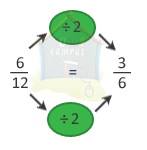We can simplify the fraction further as below.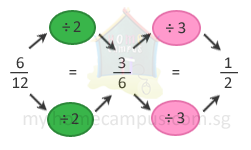The simplest form of
 6 12
is
 1 2
.

#### 2. Find the missing numerator below: 10 15 = ? 3

Compare the denominators.
15 is divided by 5 to get 3.
So, we must also divide the numerator by 5 to get a simplified equivalent fraction.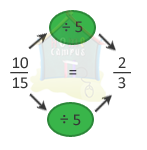10 15
=
 2 3
So, the missing numerator is 2.

#### 3. Is 4 6 the simplest equivalent fraction of 8 12 ?      If not, what is the simplest equivalent fraction of 8 12 ?

We use division to find a fraction in its simplest form.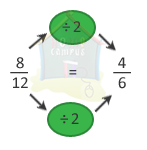We can simplify
 4 6
further.
So,
 4 6
is not the simplest equivalent fraction of
 8 12
.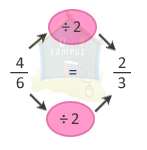The simplest equivalent fraction of
 8 12
is
 2 3
.